# Surface area of a sphere

The formula for the surface area of a sphere is:

4pir^2

We know that the area of a circle is: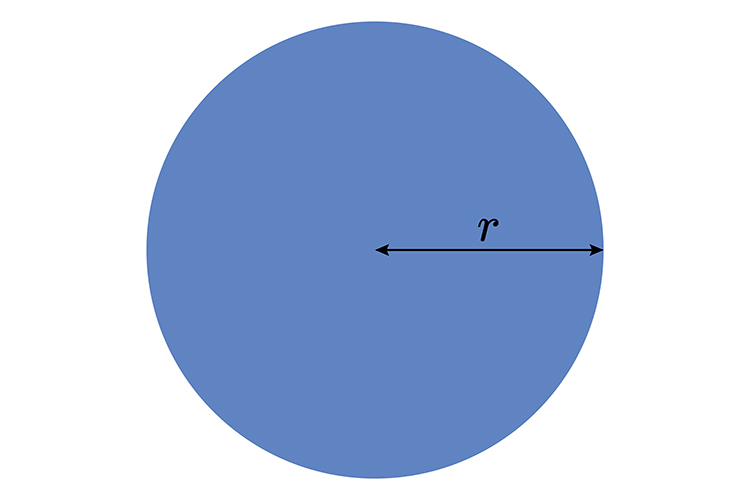Area = \pir^2

So what this is saying is that the surface area of a sphere is 4 of these.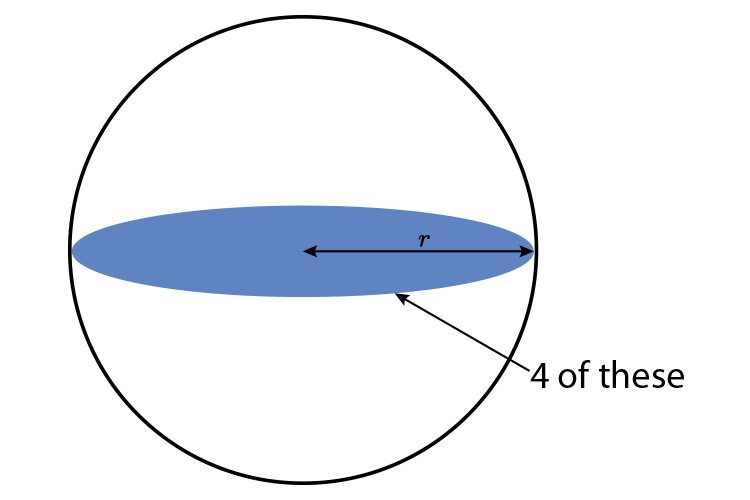So the certain way for you to ensure you never ever forget the surface area of a sphere is to carry out the orange experiment.

## The orange experiment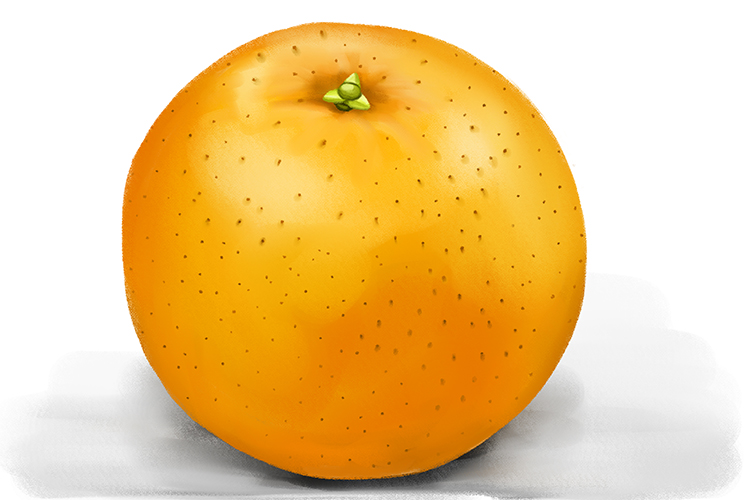The orange experiment is as follows:

Stage 1 – Take an orangeStage 2 – Cut the orange in halfStage 3 – Using one half of the orange draw four circles around it on a piece of paper.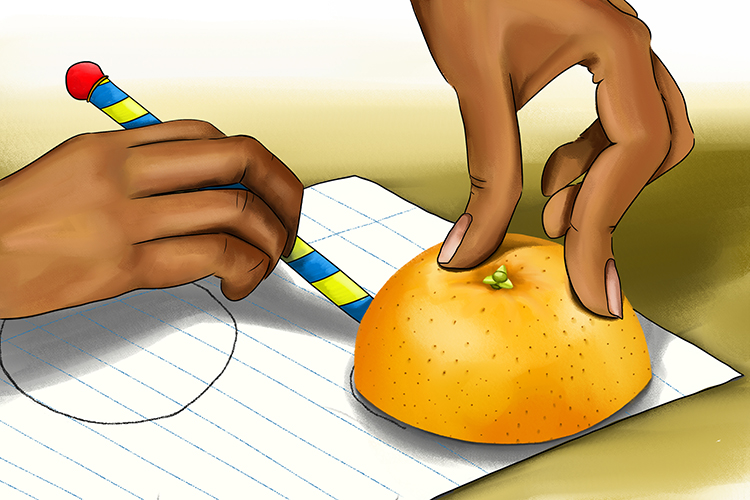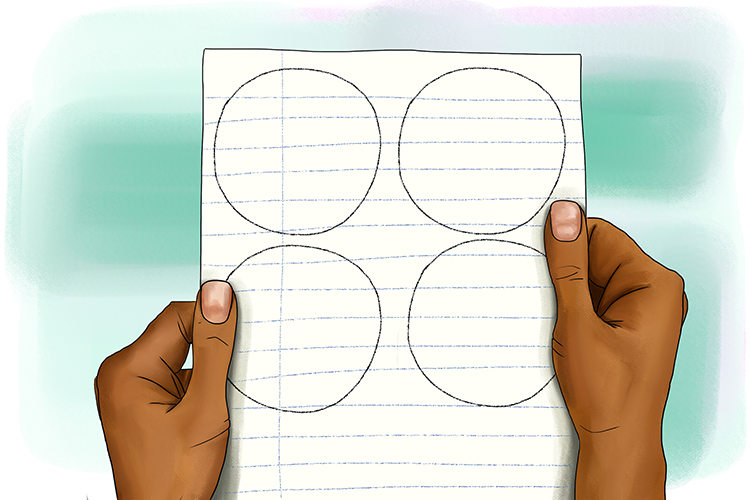Stage 4 – Now peel the orange and place the pieces in each of the circles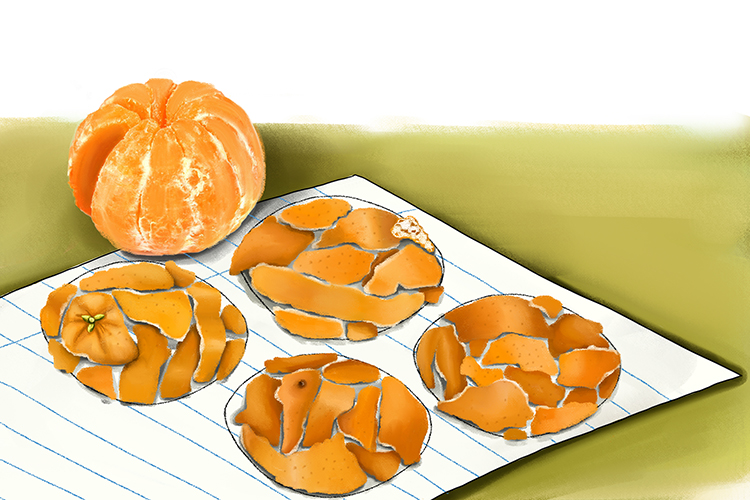Now you can see that, Yes! The surface area of an orange or any sphere really does cover four drawn out circles.

And therefore

Surface area of a sphere = 4timesArea\ of\ the\ ci\rc\l\e

Surface area of a sphere =  4\  \pir^2

Try it on the kitchen table now!